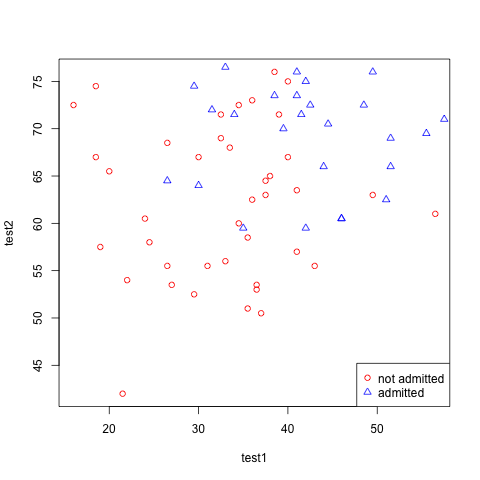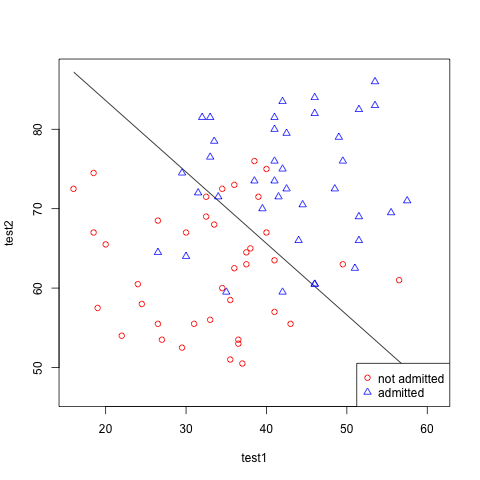# Machine Learning Ex4 – Logistic Regression and Newton’s Method

March 16, 2011
By

(This article was first published on al3xandr3, and kindly contributed to R-bloggers)

Exercise 4 is all about using Newton’s Method to implement logistic regression on a classification problem.

For all this to make sense i suggest having a look at Andrew Ng machine learning lectures on openclassroom.

We start with a dataset representing 40 students who were admitted to college and 40 students who were not admitted, and their corresponding grades for 2 exams.
Your mission, should you decide to accept it is to build a binary classification model that estimates college admission chances based on a student’s scores on two exams(test1 and test2).

## Plot the Data

We start by looking at the data.

# reading data
mydata = read.csv("http://spreadsheets.google.com/pub?key=0AnypY27pPCJydC1vRVEzM1VJQnNneFo5dWNzR1F5Umc&output=csv", header = TRUE)

# plots
plot(mydata$test1[mydata$admitted == 0], mydata$test2[mydata$admitted == 0], xlab="test1", ylab="test2", , col="red")
points(mydata$test1[mydata$admitted == 1], mydata$test2[mydata$admitted == 1], col="blue", pch=2)
legend("bottomright", c("not admitted", "admitted"), pch=c(1, 2), col=c("red", "blue") )## A Bit of Theory

Most of the ideas explored in linear regression apply in same way, first we define what the hypothesis equation looks like(the mathematical representation of this knowledge).
It originates from the line equation, but has now evolved into a new equation that returns values between [0,1] suited for binary classification. That is, we made up an equation that given test1 value and test2 value, will return the probability that the student will be admitted(y=1) into college:

[
h_theta(x) = g(theta^T x) = frac{1}{ 1 + e ^{- theta^T x} }
]

g is the sigmoid function. And this returns:

[
h_theta(x) = P (y=1 | x; theta)
]

Now we need to find the (theta) parameters for getting a working hypothesis equation.
To help with that search we define a cost equation, that for a given (theta) returns how far off we are compared to the sample data.

[
J(theta) = frac{1}{m} sum_{i=1}^m ((-y)log(h_theta(x)) – (1 – y) log(1- h_theta(x)) )
]

The lower the cost the better(closer to real data we get). Thus, the goal becomes to minimize the cost.

We can use Newton’s method for that. Newton’s method, similarly to gradient descent, is a way to search for the 0(minimum) of the derivative of the cost function. And after doing some math, the iterative (theta) updates using Newton’s method is defined as:

[
theta^{(t+1)} = theta^{(t)} – H^{-1} nabla_{theta}J
]

And the gradient and Hessian are defined like so(in vectorized versions):

[
nabla_{theta}J = frac{1}{m} sum_{i=1}^m (h_theta(x) – y) x
]

[
H = frac{1}{m} sum_{i=1}^m [h_theta(x) (1 – h_theta(x)) x^T x]
]

## Implementation

First we implement the above equations:

# sigmoid
g = function (z) {
return (1 / (1 + exp(-z) ))
} # plot(g(c(1,2,3,4,5,6)))

# hypothesis
h = function (x,th) {
return( g(x %*% th) )
} # h(x,th)

# cost
J = function (x,y,th,m) {
return( 1/m * sum(-y * log(h(x,th)) - (1 - y) * log(1 - h(x,th))) )
} # J(x,y,th,m)

# derivative of J (gradient)
grad = function (x,y,th,m) {
return( 1/m * t(x) %*% (h(x,th) - y))
} # grad(x,y,th,m)

# Hessian
H = function (x,y,th,m) {
return (1/m * t(x) %*% x * diag(h(x,th)) * diag(1 - h(x,th)))
} # H(x,y,th,m)


Make it go (iterate until convergence):

# setup variables
j = array(0,c(10,1))
m = length(mydata$test1) x = matrix(c(rep(1,m), mydata$test1, mydata$test2), ncol=3) y = matrix(mydata$admitted, ncol=1)
th = matrix(0,3)

# iterate
# note that the newton's method converges fast, 10x is enough
for (i in 1:10) {
j[i] = J(x,y,th,m) # stores each iteration Cost
th = th - solve(H(x,y,th,m)) %*% grad(x,y,th,m)
}


Have a look at the cost function by iteration:

plot(j, xlab="iterations", ylab="cost J")See that the number of iterations needed is only 4-5, converges much faster than gradient descent.

Exercise questions:

# 1. What values of  did you get? How many iterations were required for convergence?
print("1.")
print(th)

# 2. What is the probability that a student with a score of 20 on Exam 1
# and a score of 80 on Exam 2 will not be admitted?
print("2.")
print((1 - g(c(1, 20, 80) %*% th))* 100)

 "1."
[,1]
[1,] -16.4469479
[2,]   0.1457278
[3,]   0.1618285
 "2."
[,1]
[1,] 64.24722


To visualize the fit, an important remark is that: (P(y=1 | x ;theta) = 0.5) that happens when (theta^T x = 0), so:

# when ax0 + bx2 + cx3 = 0 is the middle(decision boundary line),
# so given x1 from sample data, solving to x2, we get:
x2 = (-1/th[3,]) * ((th[2,] * x1) + th[1,])

# get 2 points (that will define a line)
x1 = c(min(x[,2]), max(x[,2]))

# plot
plot(x1,x2, type='l',  xlab="test1", ylab="test2")
points(mydata$test1[mydata$admitted == 0], mydata$test2[mydata$admitted == 0], col="red")
points(mydata$test1[mydata$admitted == 1], mydata$test2[mydata$admitted == 1], col="blue", pch=2)
legend("bottomright", c("not admitted", "admitted"), pch=c(1, 2), col=c("red", "blue") )Beautiful.

#### Notes & References:

To leave a comment for the author, please follow the link and comment on their blog: al3xandr3.

R-bloggers.com offers daily e-mail updates about R news and tutorials on topics such as: Data science, Big Data, R jobs, visualization (ggplot2, Boxplots, maps, animation), programming (RStudio, Sweave, LaTeX, SQL, Eclipse, git, hadoop, Web Scraping) statistics (regression, PCA, time series, trading) and more...

If you got this far, why not subscribe for updates from the site? Choose your flavor: e-mail, twitter, RSS, or facebook...

Tags: , , ,

Comments are closed.

# Never miss an update! Subscribe to R-bloggers to receive e-mails with the latest R posts.(You will not see this message again.)

Click here to close (This popup will not appear again)